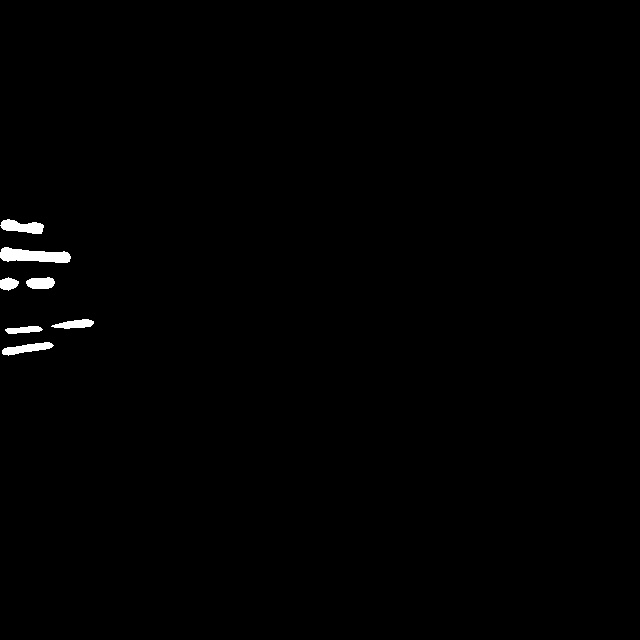``````vector<vector<cv::Mat>> map(bottom->num(), vector<cv::Mat>(bottom->channels(), cv::Mat(bottom->height(), bottom->width(), CV_32S, cv::Scalar(0))));
BlobToMat(*bottom, map);

template <typename Dtype>
void BlobToMat(const Blob<Dtype>& blob, vector<vector<cv::Mat>>& map) {
CHECK_EQ(blob.num(),1)<<"Phase:TEST, batch_size should be 1.";
const int batch_size = blob.num();
const int channel = blob.channels();
const int height = blob.height();
const int width = blob.width();
for(int i=0; i<batch_size; ++i)
for(int j=0; j<channel; ++j)
for(int m=0; m<height; ++m)
for(int n=0; n<width; ++n) {
map[i][j].at<int>(m,n) = (int)(blob.cpu_data()[((i*channel+j)*height+m)*width+n]);
}
for(int i=0; i<batch_size; ++i)
for(int j=0; j<channel; ++j) {
cv::imshow("test", map[i][j]*255*255);
cv::waitKey(0);
}
}
``````1个回答Opencv里vector<Mat>的问题

OpenCV中對vector<Mat>添加return返還

OpenCV中Mat像素访问和赋值的问题

opencv初学者提问：如何修改Mat元素的值

malloc(): memory corruption (fast): C++ opencv编写出错

c++中用openCV的IplImage*或Mat读取图片后如何转化为File文件流（或者二进制数据）的格式

arm opencv mat内存无法释放

OpenCV 的 Mat 内存错误？

OpenCV Mat对象使用数组数据块初始化
OpenCV的官网介绍Mat对象，共列出了25种基本的初始化方法。 其中，对于使用数组数据块的初始化方法官方给出的例子是 ``` double m = {{a, b, c}, {d, e, f}, {g, h, i}}; Mat M = Mat(3, 3, CV_64F, m).inv(); ``` _但是我这样初始化我的Mat对象，数据为什么不对呢 double m[] = {{1, 2, 3}, {3, 0, 9}, {1, 3, 8}}; Mat M(3, 3, CV_8U, m); 这样的方式初始化的Mat对象数据根本就不是m数组里面的数据啊。请教高手解答啊 _

1.利用opencv分别生成3507*2480的蓝(B170，G91，R4)、绿(B126，G162，R73)、红(B32，G23，R228)图片各一张。 2.利用opencv识别以上三张图片上的颜色，识别到蓝色返回B，绿色返回G，红色返回R。

``` #include<iostream> #include<opencv2/opencv.hpp> #include<stdlib.h> using namespace cv; using namespace std; // 定义全局变量 cv::Mat img_Original; cv::Mat img_Ranged; cv::Mat img_Median; // 定义回调函数 void on_low_height_thresh_trackbar(int, void*); void on_high_height_thresh_trackbar(int, void*); int low_height = 50, high_height = 85; int main() { img_Original = cv::imread("ROI1.jpg", 0); cv::medianBlur(img_Original, img_Median, 5); // 创建窗口 cv::namedWindow("original image"); cv::namedWindow("selected image"); // 创建回调函数进度条 cv::createTrackbar("Low height", "selected image", &low_height, 255, on_low_height_thresh_trackbar); cv::createTrackbar("High height", "selected image", &high_height, 255, on_high_height_thresh_trackbar); // 显示图像 cv::imshow("original image", img_Original); on_low_height_thresh_trackbar(low_height, 0); on_high_height_thresh_trackbar(high_height, 0); cv::waitKey(0); return 0; } // 定义回调函数 void on_low_height_thresh_trackbar(int, void*) { low_height = cv::min(high_height - 1, low_height); cv::setTrackbarPos("Low height", "selected image", low_height); // 选取阈值 cv::inRange(img_Median, low_height, high_height, img_Ranged); // 显示图像 cv::imshow("selected image", img_Ranged); } void on_high_height_thresh_trackbar(int, void*) { high_height = cv::max(high_height, low_height + 1); cv::setTrackbarPos("High height", "selected image", high_height); // 选取阈值 cv::inRange(img_Median, low_height, high_height, img_Ranged); // 显示图像 cv::imshow("selected image", img_Ranged); } ``` void on_low_height_thresh_trackbar(int, void*) void on_high_height_thresh_trackbar(int, void*) 在这两个函数中int，void*这两个形参都没有出现，那么设置这两个形参是为什么呢？

c++利用vector时，它是有数据头和实际存储空间二部分的，那么它是不是与opencv的Mat类差不多呢，vector<int> *f；与vector<int> f，然后在f.resize（100）或者（*f）.resize（100）这二种方法在内存的管理上是不是相差不大呢？
OpenCV Mat问题，想把数组加到矩阵的某一行，赋值出现错误
for(int i=a;i<10;) { //想把数组加到矩阵的某一行 i++; float *p; p=colsmean;//colsmean是个float数组 for(int j=0;j<64;j++) { //matsurfdes_addrow是一个Mat矩阵 matsurfdes_addrow.ptr<float>(i)[j]=(*p); p++; } } matsurfdes_addrow.copyTo(matsurfdes); //错误就出在这里，内存溢出，不知为什么，希望好心传道解惑，先行感谢！

Java学习的正确打开方式

Spring Boot2 系列教程(十七)SpringBoot 整合 Swagger2

Python 基础（一）：入门必备知识

Python十大装B语法
Python 是一种代表简单思想的语言，其语法相对简单，很容易上手。不过，如果就此小视 Python 语法的精妙和深邃，那就大错特错了。本文精心筛选了最能展现 Python 语法之精妙的十个知识点，并附上详细的实例代码。如能在实战中融会贯通、灵活使用，必将使代码更为精炼、高效，同时也会极大提升代码B格，使之看上去更老练，读起来更优雅。 1. for - else 什么？不是 if 和 else 才

2019年11月中国大陆编程语言排行榜
2019年11月2日，我统计了某招聘网站，获得有效程序员招聘数据9万条。针对招聘信息，提取编程语言关键字，并统计如下： 编程语言比例 rank pl_ percentage 1 java 33.62% 2 c/c++ 16.42% 3 c_sharp 12.82% 4 javascript 12.31% 5 python 7.93% 6 go 7.25% 7

2020 网络课 智慧树自动刷课代码，自动跳转，自动答题并关闭弹窗，自动1.5倍速静音

SQL-小白最佳入门sql查询一

“狗屁不通文章生成器”登顶GitHub热榜，分分钟写出万字形式主义大作

IT界知名的程序员曾说：对于那些月薪三万以下，自称IT工程师的码农们，其实我们从来没有把他们归为我们IT工程师的队伍。他们虽然总是以IT工程师自居，但只是他们一厢情愿罢了。 此话一出，不知激起了多少(码农)程序员的愤怒，却又无可奈何，于是码农问程序员。 码农：你知道get和post请求到底有什么区别？ 程序员：你看这篇就知道了。 码农：你月薪三万了？ 程序员：嗯。 码农：你是怎么做到的? 程序员：

《程序人生》系列-这个程序员只用了20行代码就拿了冠军

8年经验面试官详解 Java 面试秘诀
作者 | 胡书敏 责编 | 刘静 出品 | CSDN（ID：CSDNnews） 本人目前在一家知名外企担任架构师，而且最近八年来，在多家外企和互联网公司担任Java技术面试官，前后累计面试了有两三百位候选人。在本文里，就将结合本人的面试经验，针对Java初学者、Java初级开发和Java开发，给出若干准备简历和准备面试的建议。   Java程序员准备和投递简历的实

1.两种思维方式在求职面试中，经常会考察这种问题：北京有多少量特斯拉汽车？ 某胡同口的煎饼摊一年能卖出多少个煎饼？ 深圳有多少个产品经理？ 一辆公交车里能装下多少个乒乓球？ 一